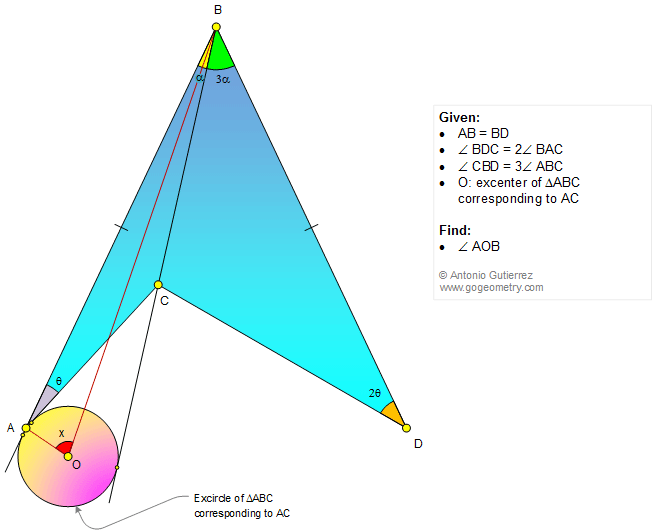# Infographic Geometry Problem 1174: Triangle, Quadrilateral, Double, Triple, Angle, Congruence, Excenter, Angle Bisector. Level: High School, College, Mathematics Education

< PREVIOUS PROBLEM  |  NEXT PROBLEM >

 In the figure, AB = BD, the measure of angle BDC is double the measure of angle BAC and the measure of angle CBD is triple the measure of angle ABC. If O is the excenter of the triangle ABC corresponding to AC (see the figure), find the measure of angle AOB. Use only elementary geometry (Euclid's Elements). . See also: Artwork of Problem 1174Home | Search | Geometry | Problems | All Problems | Open Problems | Visual Index | 10 Problems | Problems Art Gallery |  Art | 1171-1180 | Triangles | Quadrilaterals | Angles | Double angle | Angle Bisector | Excenter | CongruenceLast updated: Dec 26, 2015 by Antonio Gutierrez